#SSC Reasoning Questions : Figures with Explanation Set 1

Hello Aspirants. Welcome to Online Reasoning Section in AffairsCloud.com. Here we are creating question sample in figures , which is common for all the FCI EXAM,SSC CGL,SSC CHSL,RAILWAYS and other competitive exams. /strong>

Directions for questions : In each of these questions, there are two sets of figures. The figures on the left are Problem Figures and those on the right are Answer Figures indicated by numbers (1), (2), (3), (4) and (5). Figures form a series, if they change from left to right according to the same rule. The number of the Answer Figure which would be the next figure in the series of Problem Figures is the answer. All the six figures, i.e., five Problem Figures and one Answer Figure should be considered as forming the series.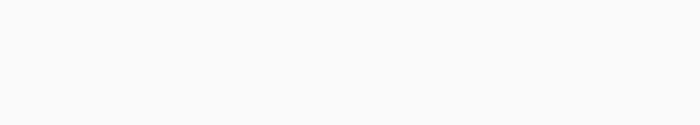ANS-(1)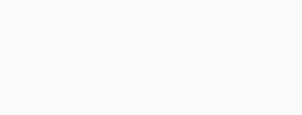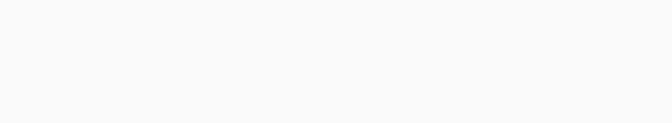Ans- 2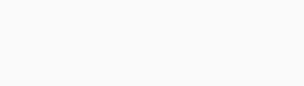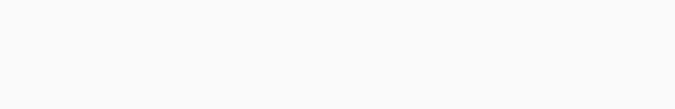ANS-4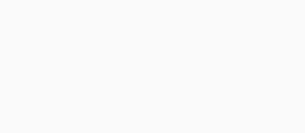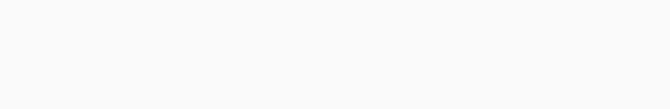ANS-5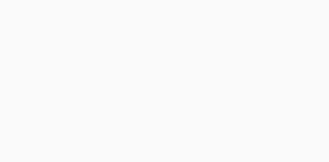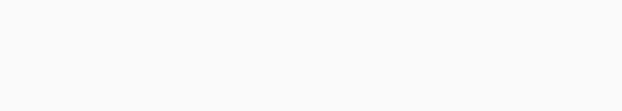ANS-3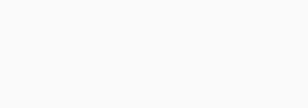Directions for questions : Among the five given figures, four figures follow the same pattern and one figure is different from these four. Mark this figure (which is different from the other four) as your answer.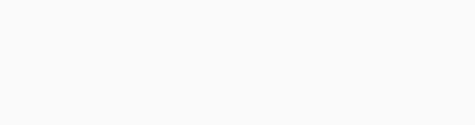ANS-4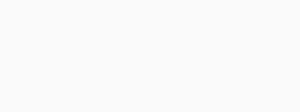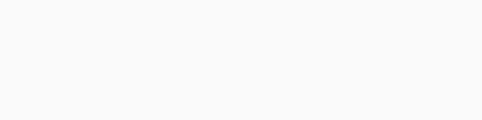ANS-3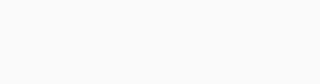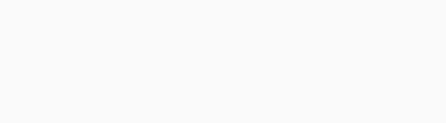ANS-5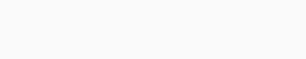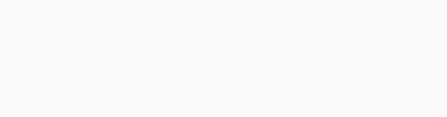ANS-3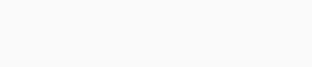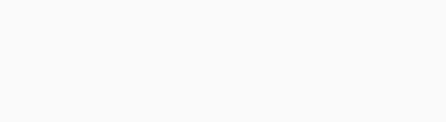ANS-3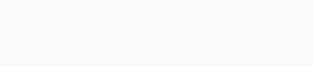AffairsCloud Ebook - Support Us to Grow